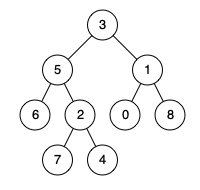# Lowest Common Ancestor of a Binary Tree

## 1: U-nderstand

Understand what the interviewer is asking for by using test cases and questions about the problem.

• Established a set (2-3) of test cases to verify their own solution later.
• Established a set (1-2) of edge cases to verify their solution handles complexities.
• Have fully understood the problem and have no clarifying questions.
• Have you verified any Time/Space Constraints for this problem?
• Are all values in tree unique?
• Yes, all values are unique.
• Can the input tree be Null?
• No, there is at least two node.
• Will p and q alway exist in tree?
• Yes, p and q node will always exist in tree
• What is the space and time complexity?
• Time is `O(N)` and Space is `O(1)` excluding the recursion stack.
``````HAPPY CASE
Input: root = [3,5,1,6,2,0,8,null,null,7,4], p = 5, q = 1
Output: 3
Explanation: The LCA of nodes 5 and 1 is 3.````````````Input: root = [3,5,1,6,2,0,8,null,null,7,4], p = 5, q = 4
Output: 5
Explanation: The LCA of nodes 5 and 4 is 5, since a node can be a descendant of itself according to the LCA definition.````````````EDGE CASE
Input: root = [1,2], p = 1, q = 2
Output: 1``````

## 2: M-atch

Match what this problem looks like to known categories of problems, e.g. Linked List or Dynamic Programming, and strategies or patterns in those categories.

If you are dealing with Binary Trees some common techniques you can employ to help you solve the problem:

• Think about appropriate Tree Traversal: Pre-Order, In-Order, Post-Order, Level-Order
• Choosing a specific tree traversal helps identify a existant node.
• Store nodes within a HashMap to refer to later
• We don’t have a specific way of referring to previous nodes in a path that could be used in a HashMap. So, a HashMap would not help us as much in this context.
• Using Binary Search to find an element
• We are not working with a Binary Search Tree.
• Applying a level-order traversal with a queue
• Using this approach may complicate our code

## 3: P-lan

Plan the solution with appropriate visualizations and pseudocode.

General Idea: Recursively, lets pass information to parent as to whether it's a left child or right child has p or q node present. When we find a parent with both p and q node present, that is the Lowest Common Ancestor, because we learn recursively that is the first node that can branches out to p and q.

``````1. Recursively inform parent as to p or q children is available to it.
a. Basecase: When we find p, q, or reach None, return the root. This lets the parent know p, q, or None children is available to it.
b. Recursively check left side and right side
c. If both left and right is available, then we have found it. Return the current root.
d. Otherwise let parent know that what we have currently, because the LCA is higher up the tree``````

⚠️ Common Mistakes

• Choosing the wrong traversal type
• Try to walk through the problem by hand and see the order in which you are processing the nodes. This will clue you into the type of traversal necessary
• Suppose you chose to perform level-order traversal, how would you determine the lowest common ancestor?

## 4: I-mplement

Implement the code to solve the algorithm.

``````# Definition for a binary tree node.
# class TreeNode:
#     def __init__(self, x):
#         self.val = x
#         self.left = None
#         self.right = None

class Solution:
# Recursively inform parent as to p or q children is available to it.
def lowestCommonAncestor(self, root: 'TreeNode', p: 'TreeNode', q: 'TreeNode') -> 'TreeNode':
# Basecase: When we find p, q, or reach None, return the root. This lets the parent know p, q, or None children is available to it.
if not root or p == root or q == root:
return root

# Recursively check left side and right side
left = self.lowestCommonAncestor(root.left, p, q)
right = self.lowestCommonAncestor(root.right,p, q)

# If both left and right is available, then we have found it. Return the current root.
if left and right:
return root

# Otherwise let parent know that what we have currently, because the LCA is higher up the tree
return left if left else right``````
``````class Solution {
public TreeNode lowestCommonAncestor(TreeNode root, TreeNode p, TreeNode q) {
// Basecase: When we find p, q, or reach None, return the root. This lets the parent know p, q, or None children is available to it.
if(root == null || root == p || root == q) return root;

// Recursively check left side and right side
TreeNode l = lowestCommonAncestor(root.left, p, q);
TreeNode r = lowestCommonAncestor(root.right, p, q);

// If both left and right is available, then we have found it. Return the current root.
if(l!=null && r!=null) return root;

// Otherwise let parent know that what we have currently, because the LCA is higher up the tree
else
{
if(l!=null) return l;
else return r;
}
}
}``````

## 5: R-eview

Review the code by running specific example(s) and recording values (watchlist) of your code's variables along the way.

• Trace through your code with an input to check for the expected output
• Catch possible edge cases and off-by-one errors

## 6: E-valuate

Evaluate the performance of your algorithm and state any strong/weak or future potential work.

Assume `N` represents the number nodes in tree

• Time Complexity: `O(N)` because we need to visit each node in binary tree.
• Space Complexity: `O(1)` if we do not count the recursion stack. The recursion stack will cost us `O(N)` if we have a linked list like tree. `O(logN)` in the average case.